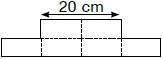# User Forum

Subject :NSO    Class : Class 4

The given figure is made up of 6 identical rectangles. If its perimeter is 108 cm, find the breadth of each rectangle.A 28 cm
B 14 cm
C 7 cm
D 10 cm

Class : Class 4

Class : Class 4

Class : Class 4

## Ans 4:

Class : Class 7
c. the length of an rectangle is 10 so the length of all the rectangles (perimeter only) is 80. 80-108=28. 28÷4=7.

Class : Class 4
P

Class : Class 5